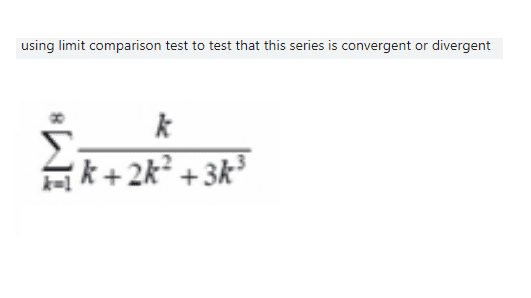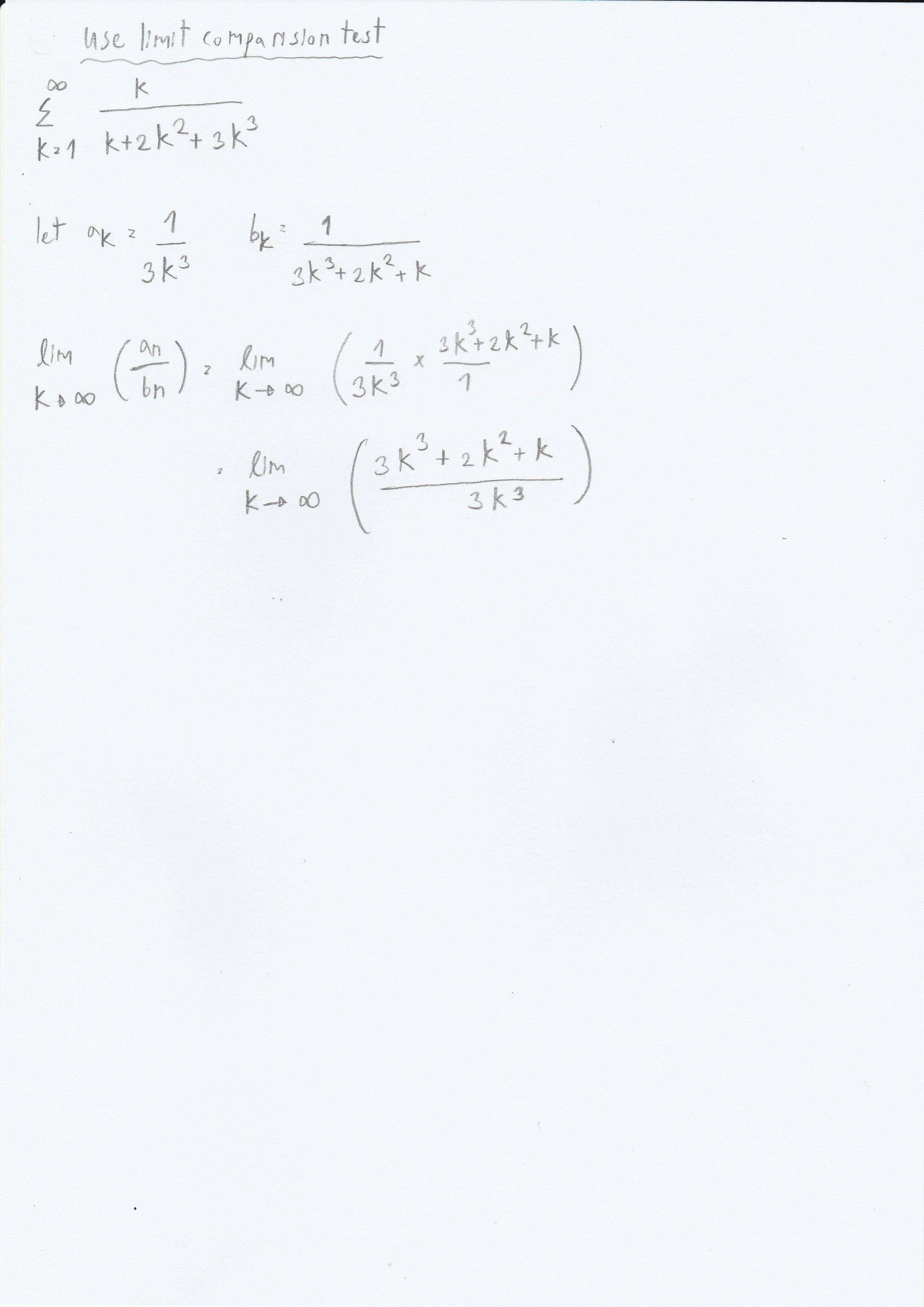# Limit comparision test

• MHB
• jaychay
The conclusion of the implication is that if $\lim a_k/b_k=c$ and $0<c<\infty$, then the answer for $\sum b_k$ is the same as the one for $\sum a_k$.f

#### jaychayHave you tried to apply the limit comparison test or find the limit of the terms as $k\to\infty$?

Have you tried to apply the limit comparison test or find the limit of the terms as $k\to\infty$?
okay I will try to do it from what you guide me and I will let you check it for me.

Have you tried to apply the limit comparison test or find the limit of the terms as $k\to\infty$?I am struggle at this point sir

Why didn't you include the numerator $k$ in $a_k$ and $b_k$? Your $b_k$ is different from the series in the problem statement.

I suggest using $a_k=1/k^2$ and $b_k$ as in the original series. Also use the following rule. If $f(x)=ax^m+\sum_{i=0}^{m-1}a_ix^i$ and $g(x)=bx^n+\sum_{i=0}^{n-1}b_ix^i$, then
$$\lim_{x\to\infty}\frac{f(x)}{g(x)}=\begin{cases}0,&m<n\\a/b,&m=n.\\\infty,&m>n\end{cases}$$

Why didn't you include the numerator $k$ in $a_k$ and $b_k$? Your $b_k$ is different from the series in the problem statement.

I suggest using $a_k=1/k^2$ and $b_k$ as in the original series. Also use the following rule. If $f(x)=ax^m+\sum_{i=0}^{m-1}a_ix^i$ and $g(x)=bx^n+\sum_{i=0}^{n-1}b_ix^i$, then
$$\lim_{x\to\infty}\frac{f(x)}{g(x)}=\begin{cases}0,&m<n\\a/b,&m=n.\\\infty,&m>n\end{cases}$$
Can you guide me more because I am very confused right now ?

The limit comparison test says that in order to ascertain convergence of $\sum b_k$ one can come up with another series $\sum a_k$ for which it is known whether it converges. If $\lim a_k/b_k=c$ and $0<c<\infty$, then the answer for $\sum b_k$ is the same as the one for $\sum a_k$.

I suggest considering $\sum b_k$ to be the series from the problem statement and $a_k=1/k^2$. It is known that $\sum 1/k^2$ converges. What limit do we have to consider to determine the convergence for $\sum b_k$?

note ...

$\dfrac{k}{k+2k^2+3k^3} = \dfrac{1}{1+2k+3k^2} < \dfrac{1}{k^2} \text{ for all } k > 1$

$\displaystyle \implies \sum_{k=1}^\infty \dfrac{1}{1+2k+3k^2} \text{ converges by direct comparison to the known convergent series } \sum_{k=1}^\infty \dfrac{1}{k^2}$

The limit comparison test says that in order to ascertain convergence of $\sum b_k$ one can come up with another series $\sum a_k$ for which it is known whether it converges. If $\lim a_k/b_k=c$ and $0<c<\infty$, then the answer for $\sum b_k$ is the same as the one for $\sum a_k$.

I suggest considering $\sum b_k$ to be the series from the problem statement and $a_k=1/k^2$. It is known that $\sum 1/k^2$ converges. What limit do we have to consider to determine the convergence for $\sum b_k$?
So here is the work that I have done recently so how can I determine that it is convergent or divergent ?
It is known that $\sum 1/k^2$ converges.
In fact, $\sum_{k=1}^\infty 1/k^2=\pi^2/6$ (see Wikipedia).
In fact, $\sum_{k=1}^\infty 1/k^2=\pi^2/6$ (see Wikipedia).
The limit comparison test is a theorem that has the following form: "For every two series $\sum a_k$ and $\sum b_k$, if ..., then ...". What is the conclusion of this implication, i.e., what is written after "then"?